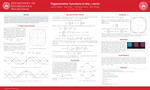## Mathematics

Squigonometry

4-2021

Presentation

Group

Mathematics

Sunil Chebolu

Mathematics

#### Abstract

Trigonometry is the study of circular functions - functions defined on the unit circle where distances are measured with respect to the Euclidean norm. In our research, we develop a parallel theory of trigonometric and inverse trigonometric functions for the p-norm. This is called squigonometry because the resulting functions are defined on a squircle. This approach leads to new transcendental periods, formulas, and identities. It also extends to exponential, hyperbolic, and logarithmic functions in the p-norm.

#### Notes

Authors: Sunil Chebolu, Andrew Hatfield, Riley Klette, Christopher Moore, Beth WardenCOinS